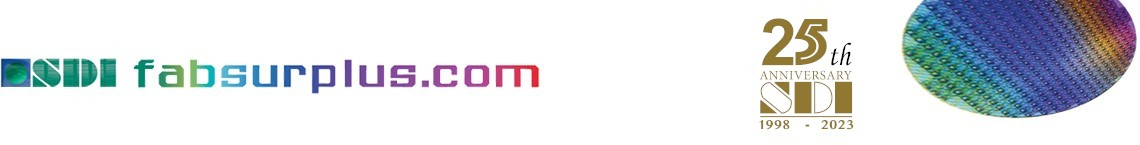List of Daihen equipment available for sale at fabsurplus.com

The following are the items available for sale related to Daihen at SDI fabsurplus.com. To inquire about the Daihen equipment item you need, click on the relevant link below to get more details, and inquiry if interested. If no result is shown, please try to search for another item or inquiry us about your request of Daihen items.

SDI ID Manufacturer Model Description Version Vintage Q. ty Sales Conditions Lead Time
89388 Daihen RNM-50N6 Match Spares 1 inquire
89390 Daihen DAUMA-10M2H Match Spares 1 inquire
89391 Daihen Controller Spares 1 inquire
89392 Daihen RNM-20E2 Match Spares 1 inquire
89393 Daihen RMN-40A Match Spares 1 inquire
89394 Daihen MFM20SA2 Match Spares 1 inquire
82012 DAIHEN CB-13B ETCH 1 as is where is
82013 DAIHEN HMN-30A ETCH 29 as is where is
82014 DAIHEN HRM-30B ETCH 1 as is where is
82015 DAIHEN HRM-30B1 ETCH 1 as is where is
82016 DAIHEN RMN-50X ETCH 30 as is where is
93284 Daihen ATP-10A1 RF GENERATOR SPARES 1 as is where is
93285 Daihen DCP-208-24 RF GENERATOR SPARES 1 as is where is
95388 Daihen RGA-150A1 RF GENERATOR, 13MHz to 14 MHz, 15 KW Spares 01.12.2008 1 as is where is immediately
82090 DAIHEN 15Z-TW1 ETCH 1 as is where is
82091 DAIHEN AGA-50B2 ETCH 9 as is where is
82092 DAIHEN ATP-30B ETCH 1 as is where is
82093 DAIHEN FGA-30C ETCH 2 as is where is
82094 DAIHEN HGA-30A ETCH 30 as is where is
82095 DAIHEN HGA-30B1 ETCH 2 as is where is
82096 DAIHEN RGA-50F ETCH 30 as is where is
82097 DAIHEN RGA-50G1 ETCH 1 as is where is
82098 DAIHEN RTGA-30A1 ETCH 1 as is where is
82099 DAIHEN WGA-50E ETCH 13 as is where is
88001 DAIHEN AGA-50B2 RF Generator Spares 6 as is where is
88002 DAIHEN AMN-30F RF Generator Spares 1 as is where is
88003 DAIHEN AMN-50B2 RF Generator Spares 2 as is where is
88004 DAIHEN AMN-50B2A RF Generator Spares 3 as is where is
88005 DAIHEN AMN-50F RF Generator Spares 10 as is where is
88006 DAIHEN AMN-50F RF Generator Spares 1 as is where is
88007 DAIHEN ATM-30A RF Generator Spares 1 as is where is
88008 DAIHEN ATP-15B RF Generator Spares 2 as is where is
88009 DAIHEN ATP-30B RF Generator Spares 1 as is where is
88010 DAIHEN FRM-30A RF Generator Spares 9 as is where is
88011 DAIHEN FRM-30A2 RF Generator Spares 6 as is where is
88012 DAIHEN FTM-80A1 RF Generator Spares 19 as is where is
88013 DAIHEN HFA-27B RF Generator Spares 1 as is where is
88014 DAIHEN HGA-30B RF Generator Spares 4 as is where is
88015 DAIHEN HRM-30B4 RF Generator Spares 1 as is where is
88016 DAIHEN MFG-20SA3 RF Generator Spares 2 as is where is
88017 DAIHEN NX-PLB-01 RF Generator Spares 3 as is where is
88018 DAIHEN RGA-10G RF Generator Spares 3 as is where is
88019 DAIHEN RMN-50M RF Generator Spares 2 as is where is
88020 DAIHEN RTGA-30A1 RF Generator Spares 18 as is where is
88021 DAIHEN WGA-50E RF Generator Spares 10 as is where is
88022 DAIHEN WGA-50E1 RF Generator Spares 1 as is where is
88023 DAIHEN WMN-50C6A RF Generator Spares 2 as is where is
88024 DAIHEN WMN-50G RF Generator Spares 2 as is where is